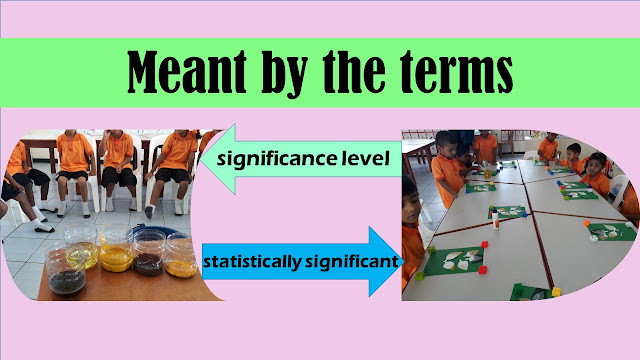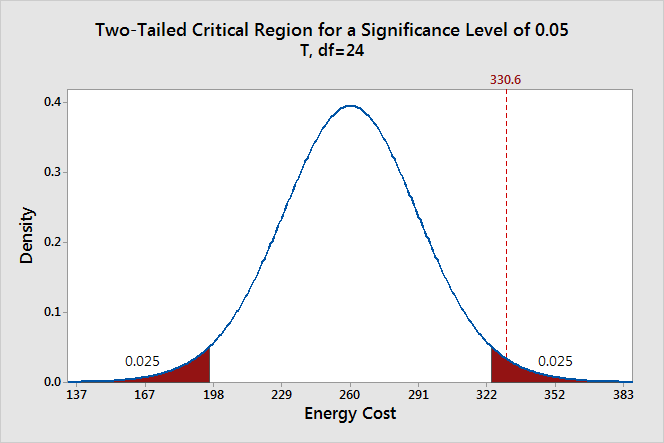# Significance level and Statistically significant

### October 31, 2019State what is meant by the terms "significance level" and "statistically significant."
The level at which one can accept whether an event is statistically significant is known as the significance level. Researchers use a test statistic known as the p-value to discern whether the event falls below the significance level; if it does, the result is statistically significant. In statistics, the p-value is the probability of obtaining the observed results of a test, assuming that the null hypothesis is correct. The p-value is used as an alternative to rejection points to provide the smallest level of significance at which the null hypothesis would be rejected.

The significance level also denoted as alpha or α, is a measure of the strength of the evidence that must be present in your sample before you will reject the null hypothesis and conclude that the effect is statistically significant. The researcher determines the significance level before conducting the experiment.
Statistical Significance. What Is Statistical Significance?
Statistical significance refers to the claim that a result of data generated by testing or experimentation is not likely to occur randomly or by chance but is instead likely to be attributable to a specific cause. Having statistical significance is important for academic disciplines or practitioners that rely heavily on analyzing data and research, such as economics, finance, investing, medicine, physics, and biology. Statistical significance can be considered strong or weak. When analyzing a data set and doing the necessary tests to discern whether one or more variables have an effect on an outcome, strong statistical significance helps support the fact that the results are real and not caused by luck or chance. Simply stated, if a statistic has high significance then it's considered more reliable.

Problems arise in tests of statistical significance because researchers are usually working with samples of larger populations and not the populations themselves. As a result, the samples must be representative of the population, so the data contained in the sample must not be biased in any way.Understanding Statistical Significance
The calculation of statistical significance (significance testing) is subject to a certain degree of error. The researcher must define in advance the probability of a sampling error, which exists in any test that does not include the entire population.

The sample size is an important component of statistical significance in that larger samples are less prone to flukes. Only random, representative samples should be used in significance testing. The level at which one can accept whether an event is statistically significant is known as the significance level. Researchers use a test statistic known as the p-value to discern whether the event falls below the significance level; if it does, the result is statistically significant. The p-value is a function of the means and standard deviations of the data samples.

The p-value indicates the probability under which a statistical result occurred by chance or by sampling error. In other words, the p-value indicates the risk that there is no actual difference or relationship. The p-value must fall under the significance level for the results to at least be considered statistically significant. The opposite of the significance level, calculated as 1 minus the significance level, is the confidence level. It indicates the degree of confidence that the statistical result did not occur by chance or by sampling error.
Types of Statistical Significance Tests
Several types of significance tests are used depending on the research being conducted. For example, tests can be employed for one, two, or more data samples of various size for averages, variances, proportions, paired or unpaired data, or different data distributions.
The Null Hypothesis
All these factors have what is called null hypotheses, and significance often is the goal of hypothesis testing in statistics. The most common null hypothesis is that the variable in question is equal to zero. If you can reject the null hypothesis with a confidence of 95 per cent or better, researchers can invoke statistical significance. Null hypotheses can also be tested for the equality of effect for two or more alternative treatments—for example, between a drug and a placebo in a clinical trial.FB Page

@ELITE_Mv